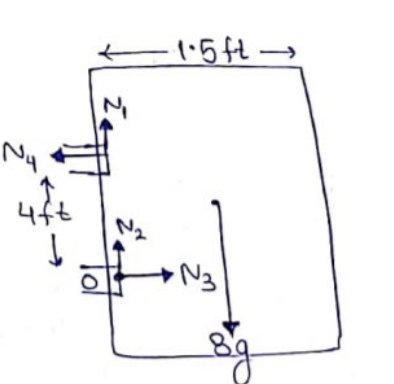# Solve the following :Question:

The door of an almirah is $6 \mathrm{ft}$ high, $1.5 \mathrm{ft}$ wide and weighs $8 \mathrm{~kg}$. The door is supported by two hinges situated at a distance of $1 \mathrm{ft}$ from the ends. If the magnitudes of the force exerted by the hinges on the door are equal, find this magnitude.

Solution:Magnitude of forces by hinges are equal.

$\sqrt{N_{1}^{2}+N_{4}^{2}}=\sqrt{N_{2}^{2}+N_{3}^{2}}$

$N_{1}^{2}+N_{4}^{2}=N_{2}^{2}+N_{3}^{2}-(\mathrm{i})$

Rotational Equilibrium at point $O$

$N_{4}(4)=8 g\left(\frac{1.5}{2}\right)$

$N_{4}=1.5 \mathrm{~g}$

Bv translational Equilibrium

$N_{3}=N_{4}=1.5 g$-(ii)

$N_{1}+N_{2}=8 g$

Solving (i),(ii),(iii)

$N_{1}=N_{2}=4 g$

Magnitude of force $=\sqrt{N_{1}^{2}+N_{4}^{2}}$

$=\sqrt{(4 g)^{2}+(1.5 g)^{2}}$

$=43 \mathrm{~N}$